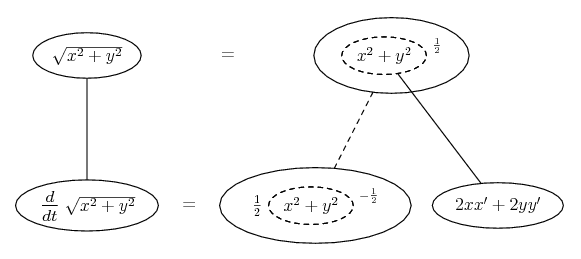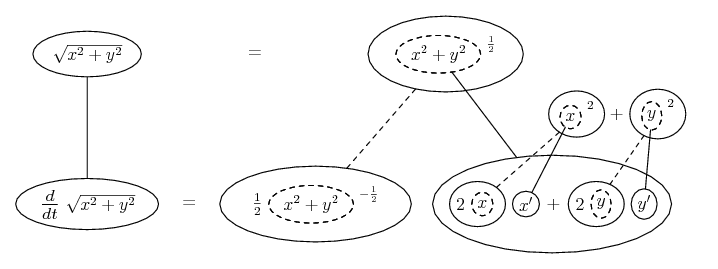# Thread: Step by step for related rates with chain rule?

1. ## Step by step for related rates with chain rule?

I have the following function in terms of time:

$\displaystyle M(t) = \sqrt{x(t)^2 + y(t)^2}$

I know the differentiation with respect to t is:

$\displaystyle M'(t) = \frac{xx' + yy'}{\sqrt{x^2 + y^2}}$

However, I don't know how to do the differentiation to get that answer. I know the chain rule needs to be used, but I don't know exactly how to use it. Could someone please help me understand? My textbook is not as clear as I had hoped, and it unfortunately skips steps.

2.Originally Posted by Redding1234I have the following function in terms of time:

$\displaystyle M(t) = \sqrt{x(t)^2 + y(t)^2}$

I know the differentiation with respect to t is:

$\displaystyle M'(t) = \frac{xx' + yy'}{\sqrt{x^2 + y^2}}$

However, I don't know how to do the differentiation to get that answer. I know the chain rule needs to be used, but I don't know exactly how to use it. Could someone please help me understand? My textbook is not as clear as I had hoped, and it unfortunately skips steps.
Write the function as

$\displaystyle M(t) = \left\{[x(t)]^2 + [y(t)]^2\right\}^{\frac{1}{2}}$

Now let $\displaystyle u = [x(t)]^2 + [y(t)]^2$ so that $\displaystyle M = u^{\frac{1}{2}}$.

$\displaystyle \frac{dM}{du} = \frac{1}{2}u^{-\frac{1}{2}}$

$\displaystyle = \frac{1}{2\sqrt{[x(t)]^2 + [y(t)]^2}}$.

To find $\displaystyle \frac{du}{dt}$, you will need to use the chain rule on each term.

So $\displaystyle \frac{du}{dt} = 2x(t)x'(t) + 2y(t)y'(t)$.

Therefore

$\displaystyle M'(t) = \frac{du}{dt}\,\frac{dM}{du}$

$\displaystyle = \frac{2x(t)x'(t) + 2y(t)y'(t)}{2\sqrt{[x(t)]^2 + [y(t)]^2}}$

$\displaystyle = \frac{x(t)x'(t) + y(t)y'(t)}{\sqrt{[x(t)]^2 + [y(t)]^2}}$.

3.Originally Posted by Redding1234I have the following function in terms of time:

$\displaystyle M(t) = \sqrt{x(t)^2 + y(t)^2}$

I know the differentiation with respect to t is:

$\displaystyle M'(t) = \frac{xx' + yy'}{\sqrt{x^2 + y^2}}$

However, I don't know how to do the differentiation to get that answer. I know the chain rule needs to be used, but I don't know exactly how to use it. Could someone please help me understand? My textbook is not as clear as I had hoped, and it unfortunately skips steps.
The derivative of $\displaystyle M(t) = \sqrt{x^2 + y^2}$ is $\displaystyle M'(t) = \frac{1}{\sqrt{x^2 + y^2}}(2x\frac{dx}{dt}+2y\frac{dy}{dt})$

The chain rule treats $\displaystyle M(t) = \sqrt{x^2 + y^2}$ as $\displaystyle \frac{1}{\sqrt{x}}$ then multiplies by the derivative of the inside with respect to t, in this case $\displaystyle (2x\frac{dx}{dt}+2y\frac{dy}{dt})$

4. Thanks so much for the replies!Originally Posted by Prove ItTo find $\displaystyle \frac{du}{dt}$, you will need to use the chain rule on each term.

So $\displaystyle \frac{du}{dt} = 2x(t)x'(t) + 2y(t)y'(t)$.
I guess the only part I'm hung up on is that. I think I was being thrown by the "dt." Nothing is actually getting differentiated by the "dt" though because we are using implicit differentiation, right?

More explicitly, is this what's going on?

$\displaystyle \frac{du}{dt} = \frac{d}{dt}[x(t)^2 + y(t)^2] = \frac{d}{dx}[x(t)^2 + y(t)^2](\frac{dx}{dt}) + \frac{d}{dy}[x(t)^2 + y(t)^2](\frac{dy}{dt})$

$\displaystyle = [2x(t) + 0]\frac{dx}{dt} + [0 + 2y(t)]\frac{dy}{dt}$

$\displaystyle = 2x(t)\frac{dx}{dt} + 2y(t)\frac{dy}{dt}$.

5.Originally Posted by Redding1234More explicitly, is this what's going on?

$\displaystyle \frac{du}{dt} = \frac{d}{dt}[x(t)^2 + y(t)^2] = \frac{d}{dx}[x(t)^2 + y(t)^2](\frac{dx}{dt}) + \frac{d}{dy}[x(t)^2 + y(t)^2](\frac{dy}{dt})$

$\displaystyle = [2x(t) + 0]\frac{dx}{dt} + [0 + 2y(t)]\frac{dy}{dt}$

$\displaystyle = 2x(t)\frac{dx}{dt} + 2y(t)\frac{dy}{dt}$.
That's fine if you really want to use the multi-variable chain rule - except it's customary then to write $\displaystyle \frac{\partial}{\partial x}$ and $\displaystyle \frac{\partial}{\partial y}$ instead of $\displaystyle \frac{d}{dx}$ and $\displaystyle \frac{d}{dy}$. However, the sum rule for differentiation allows you to apply the ordinary chain rule term by term, as Prove It was suggesting. So if you wanted to show that more explicitly it would be just...

$\displaystyle \frac{du}{dt} = \frac{d}{dt}[x(t)^2 + y(t)^2] = \frac{d}{dx}x(t)^2(\frac{dx}{dt}) + \frac{d}{dy}y(t)^2(\frac{dy}{dt})$

and from there straight to your last line.

Just in case a picture is of interest...... and, zooming in on the inner derivative...... where... is the chain rule. Straight continuous lines differentiate downwards (integrate up) with respect to t, and the straight dashed line similarly but with respect to the dashed balloon expression (the inner function of the composite which is subject to the chain rule).
_________________________________________
Don't integrate - balloontegrate!

Balloon Calculus; standard integrals, derivatives and methods

Balloon Calculus Drawing with LaTeX and Asymptote!

#### Search Tags

chain, rates, related, rule, step